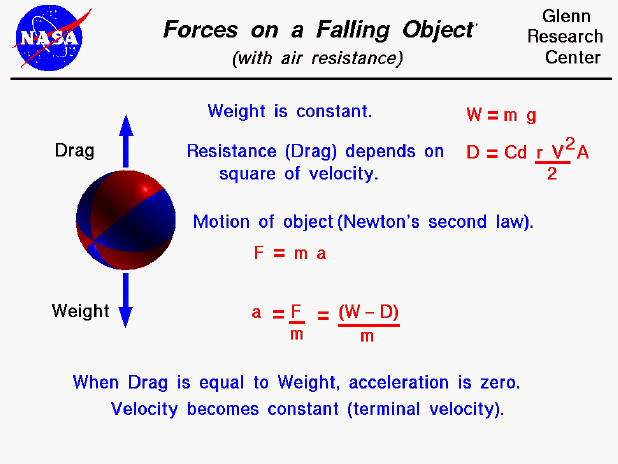An object that is falling through the atmosphere is subjected to two external forces. The first force is the gravitational force, expressed as the weight of the object. The weight equation, defines the weight (W) to be equal to the mass (m) of the object times the gravitational acceleration (g) which is 9.8 meters per square second on the surface of the earth. The gravitational acceleration decreases with the square of the distance from the center of the earth. So for most practical problems in the atmosphere, we can assume this factor to be a constant. If the object were falling in a vacuum, this would be the only force acting on the object. But in the atmosphere, the motion of a falling object is opposed by the air resistance, or drag. The drag equation tells us that drag (D) is equal to a drag coefficient (Cd) times one half the air density (r) times the velocity (V) squared times a reference area (A) on which the drag coefficient is based.

The motion of a falling object can be described by Newton's second law of motion, Force (F) = mass (m) times acceleration (a). We can do a little algebra and solve for the acceleration of the object in terms of the net external force and the mass of the object (a = F / m). The net external force is equal to the difference between the weight and the drag forces (F = W - D). The acceleration of the object then becomes a = (W - D) / m . The drag force depends on the square of the velocity. So as the body accelerates its velocity (and the drag) will increase. It will reach a point where the drag is exactly equal to the weight. When drag is equal to weight, there is no net external force on the object, and the acceleration will become equal to zero. The object will then fall at a constant velocity as described by Newton's First Law of Motion. The constant velocity is called the terminal velocity.

Guided Tours